Related Articles

# Algebraic Solutions of Linear Inequalities in One Variable and their Graphical Representation – Linear Inequalities | Class 11 Maths

Two algebraic expressions or real numbers related by the symbol ≤, ≥, < and > form an inequality. If the expressions are linear then the inequality is called linear inequality.  Eg : 4(x – 2) < 10, 10 < 45, x >= -3, etc.

Symbols of linear inequality:

• Not Equal to (≠)
• Greater Than (>)
• Less Than (<)
• Greater Than or Equal To (≥)
• Less Than or Equal To (≤)

The Greater than (>) and Less than (<) are called Strict Inequalities as they depict that a number is strictly less or more than the other.

The Greater Than or Equal To (≥) and Less Than or Equal To (≤) are called Slack Inequalities or non-strict inequalities as they depict that the value is included in the solution.

### Properties of Linear Inequality

• We can add or subtract a real number from both sides of the inequality
• We can multiply or divide both the sides of the inequality by a positive number
• If we multiply or divide both the sides of the inequality by a negative number then the inequality sign gets reversed

### Types of Linear Inequality

• Linear Inequality in one variable: If the linear function involves a single variable then it is linear inequality in one variable. Eg: 2x – 45 > 7
• Linear inequality in two variables: If the linear function involves two variables then it is linear inequality in two variables. Eg : 4x + 7y > 9

In this article, we will focus on Linear Inequalities in One Variable.

To solve linear inequality in one variable we need to follow these steps:

Step 1: Separate the constants on one side and the variables on the other side.

Step 2: Simplify both the side to convert into an equation of the form mx > n or mx < n.

Step 3: Divide both the side by m, if m is negative then reverse the inequality sign.

### Sample Problems on Linear Inequality in One Variable

Problem 1: Solve for x, if x +4 ≥ 18

Solution:

x + 4 ≥ 18

x + 4 – 4 ≥ 18 – 4

x ≥ 14

Problem 2: Solve for x, if3x ≥ 21

Solution:

= −3x ≥ 21

= x ≤ 21/-3 ( Change in sign is due to division of both sides by a negative number )

=  x ≤ −7

Problem 3: Solve for x, if 5 – 2x ≥ 19

Solution:

5 – 2x ≥ 19

= -2x ≥ 19 – 5

= -2x ≥ 14

= x ≤ 14/-2 (Change in sign is due to division by a negative number)

= x ≤ -7

Problem 4: Solve for x, if 7x + 4 < 5(x + 2)

Solution:

7x + 4 < 5(x + 2)

7x + 4 < 5x + 10

7x – 5x < 10 – 4

2x < 6

x < 3

### Graph of Linear Inequality in One Variable

As there is only one variable it will be represented on a number line. Steps for graphing a solution of a linear inequality on a number line:

Step 1: Solve the inequality

Step 2: Make a number line and plot the point on the number line

Step 3: Locate the real no. found after solving the inequality. Use an open circle for Strict Inequality(< and >) and a closed circle for Slack Inequality(≤ and ≥)

Step 4: In the final inequality if the sign is > or ≥ draw the line towards the positive axis or towards the negative axis if the sign is < or ≤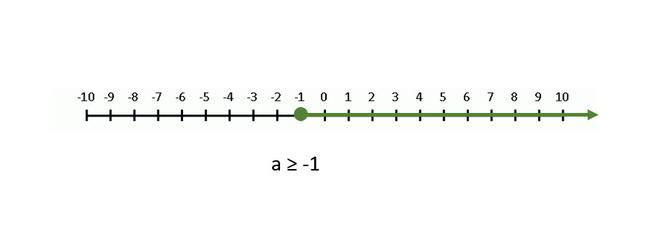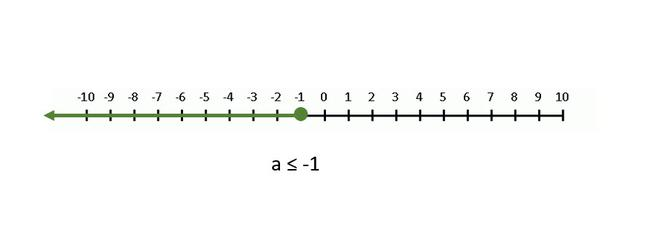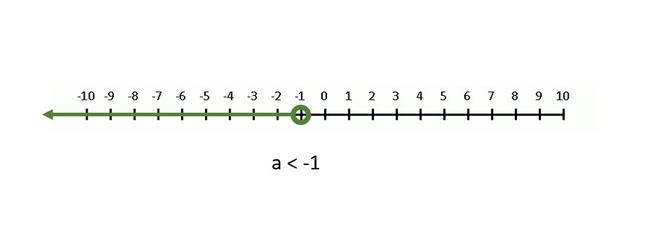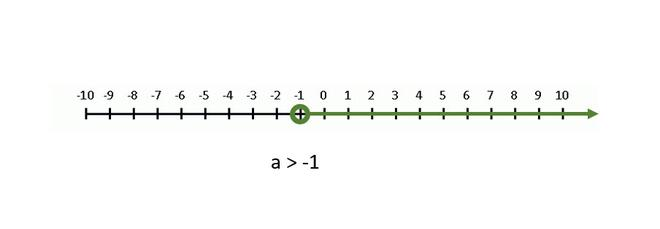### Sample Problems on Graphs

Problem 1: Solve the linear inequality (3a + 7)/2 ≥ a + 5, and represent the solution on the number line.

Solution:

(3a + 7)/2 ≥ a + 5

= 3a + 7 ≥ 2a + 10

= a ≥ 3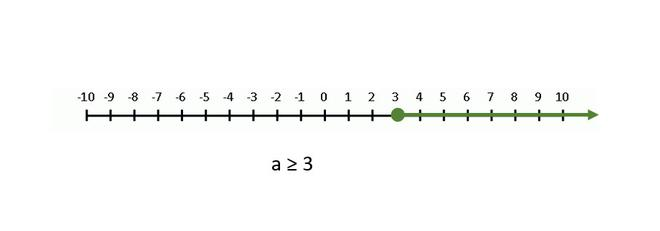Problem 2: Solve the linear inequality −13 < 3x – 7 < 17 and represent the solution on the number line.

Solution:

-13 < 3x – 7 < 17

-13 + 7 < 3x – 7 + 7 < 17 + 7

-6 < 3x < 24

-6/3 < x < 24/3

-2 < x < 8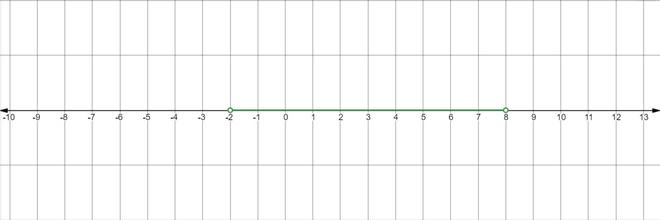### Word Problems on Linear Inequality

Problem 1: In the first four papers each of 100 marks, Devesh got 97, 75, 75, 84 marks. If he wants an average of greater than 80 marks and less than 85 marks, find the range of marks he should score in the fifth paper.

Solution:

Let x be the marks in the fifth paper. According to the statement,

80 < (97 + 75 + 75 + 84 + x)/5 < 85

= 400 < 331 + x < 425

= 400 – 331 < x < 425 – 331

= 69 < x < 94

Therefore, Devesh needs to score greater than 69 and less than 94 to get his desired average.

Problem 2: Surya has two rods. The length of one rod is three meters longer than the other, each of the rods is shorter than 19m, and the sum of the two rods is longer than 23m. Find a possible range of length of the shorter rod.

Solution:

Let the length of the shorter rod be x. Then the length of the other rod is x + 3.

In solving this kind of problem, when both the rod lengths are less than some number, we have to take the length of the longer rod to form inequality.

x + 3 < 19

x < 16

The sum of the length of the rods is more than 23,

x + x + 3 > 23

= 2x > 20

= x > 10

Thus, 10 < x < 16

Problem 3: Ola charges a Rs 20 flat rate in addition to Rs 4 per kilometer. Arnab has no more than Rs 100 to spend on a ride. How many kilometers can Arnab travel without exceeding his budget?

Solution:

Let the no of kilometer traveled by Arnab be x.

According to the statement,

4x + 20 ≤ 100

= 4x ≤ 80

= x ≤ 20

Thus, Arnab can travel 20 kilometers or less before reaching his limit of Rs 100.

Whether you're preparing for your first job interview or aiming to upskill in this ever-evolving tech landscape, GeeksforGeeks Courses are your key to success. We provide top-quality content at affordable prices, all geared towards accelerating your growth in a time-bound manner. Join the millions we've already empowered, and we're here to do the same for you. Don't miss out - check it out now!

Previous
Next